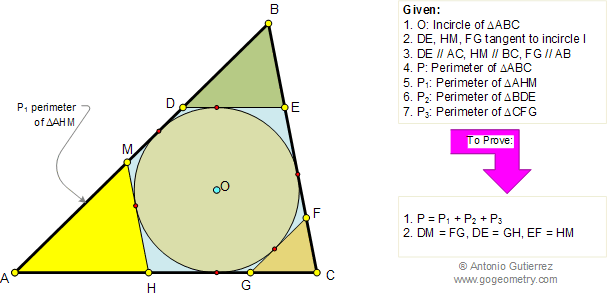# Geometry Problem 141. Triangle, Incircle, Tangent and parallel to side, Perimeter

In the figure below, given a triangle ABC and the incircle O (inscribed circle), DE, FG, and HM are tangent to the incircle O and  parallel to AC, AB, and BC respectively. If P, P1, P2, P3 are the perimeters of triangles ABC, AHM, BDE, and CFG respectively, (1) prove that P = P1 + P2 + P3, and (2) DM = FG, DE = GH, EF = HM. View or post a solution.Home | Geometry | Search | Problems | 141-150 | Semiperimeter | Email